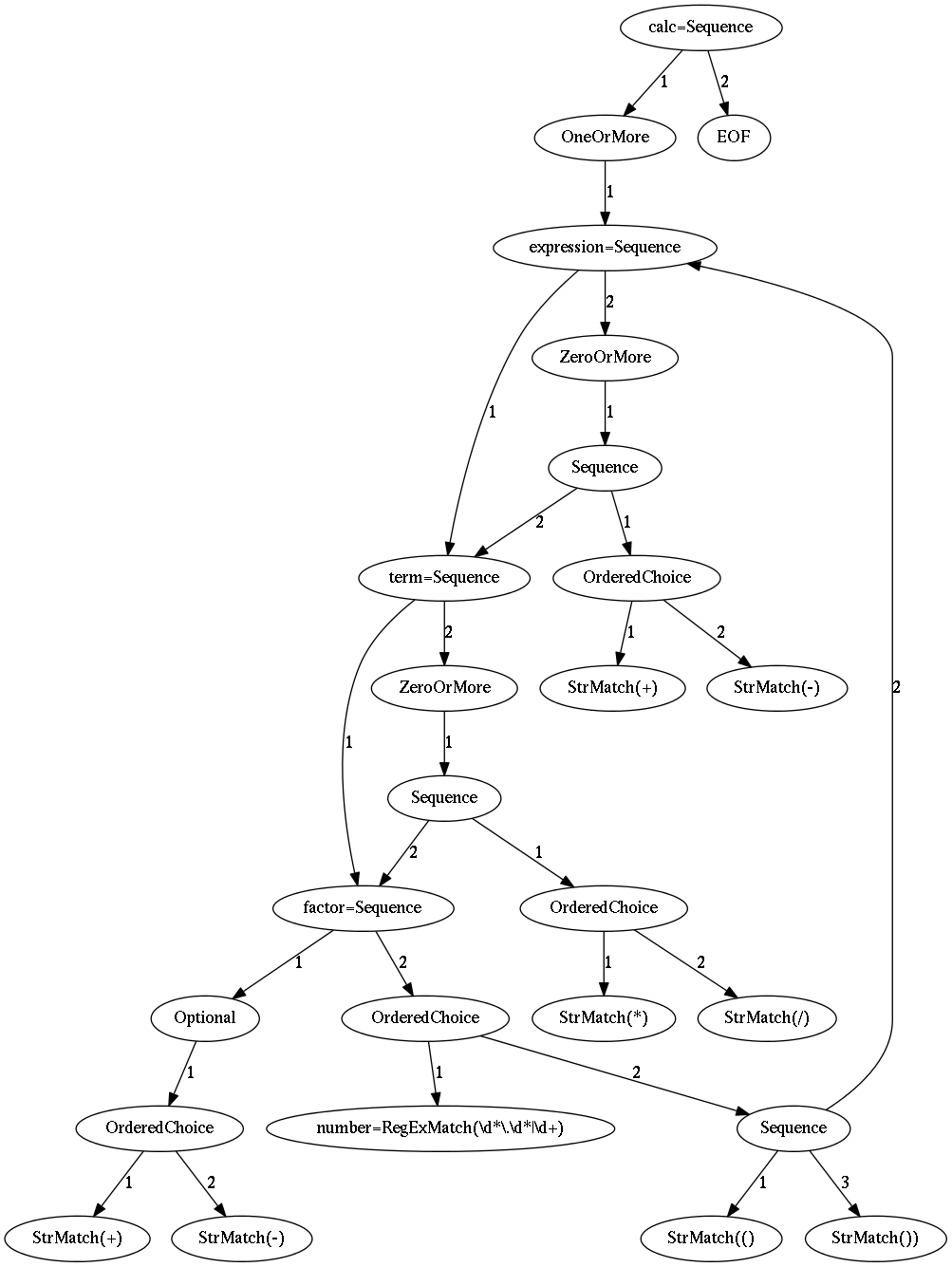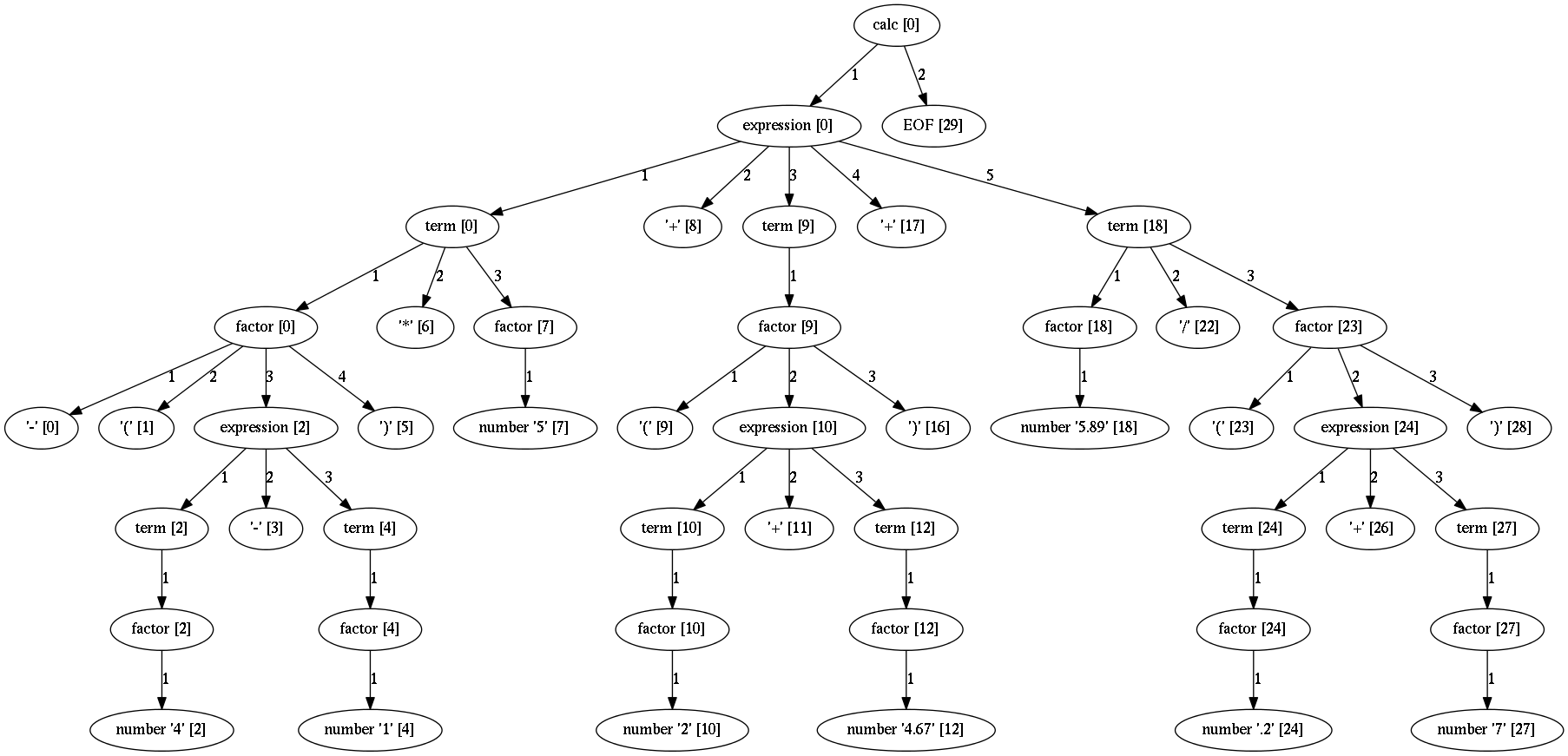# Getting started¶

Installation and your first steps with Arpeggio.

## Installation¶

Arpeggio is written in Python programming language and distributed with setuptools support. If you have `pip` tool installed the most recent stable version of Arpeggio can be installed form PyPI with the following command:

``````\$ pip install Arpeggio
``````

To verify that you have installed Arpeggio correctly run the following command:

``````\$ python -c 'import arpeggio'
``````

If you get no error, Arpeggio is correctly installed.

To install Arpeggio for contribution see here.

### Installing from source¶

If for some weird reason you don't have or don't want to use `pip` you can still install Arpeggio from source.

``````\$ wget https://github.com/textX/Arpeggio/archive/v1.1.tar.gz
``````
• unpack

``````\$ tar xzf v1.1.tar.gz
``````
• install

``````\$ cd Arpeggio-1.1
\$ python setup.py install
``````

## Quick start¶

Basic workflow in using Arpeggio goes like this:

Write a grammar. There are several ways to do that:

• The canonical grammar format uses Python statements and expressions. Each rule is specified as Python function which should return a data structure that defines the rule. For example a grammar for simple calculator can be written as:

``````from arpeggio import Optional, ZeroOrMore, OneOrMore, EOF
from arpeggio import RegExMatch as _

def number():     return _(r'\d*\.\d*|\d+')
def factor():     return Optional(["+","-"]), [number, ("(", expression, ")")]
def term():       return factor, ZeroOrMore(["*","/"], factor)
def expression(): return term, ZeroOrMore(["+", "-"], term)
def calc():       return OneOrMore(expression), EOF
``````

The python lists in the data structure represent ordered choices while the tuples represent sequences from the PEG. For terminal matches use plain strings or regular expressions.

• The same grammar could also be written using traditional textual PEG syntax like this:

``````number <- r'\d*\.\d*|\d+';  // this is a comment
factor <- ("+" / "-")? (number / "(" expression ")");
term <- factor (( "*" / "/") factor)*;
expression <- term (("+" / "-") term)*;
calc <- expression+ EOF;
``````
• Or similar syntax but a little bit more readable like this:

``````number = r'\d*\.\d*|\d+'    # this is a comment
factor = ("+" / "-")? (number / "(" expression ")")
term = factor (( "*" / "/") factor)*
expression = term (("+" / "-") term)*
calc = expression+ EOF
``````

The second and third options are implemented using canonical first form. Feel free to implement your own grammar syntax if you don't like these (see modules `arpeggio.peg` and `arpeggio.cleanpeg`).

Instantiate a parser. Parser works as a grammar interpreter. There is no code generation.

``````from arpeggio import ParserPython
parser = ParserPython(calc)   # calc is the root rule of your grammar
# Use param debug=True for verbose debugging
# messages and grammar and parse tree visualization
# using graphviz and dot
``````

``````parse_tree = parser.parse("-(4-1)*5+(2+4.67)+5.89/(.2+7)")
``````

If parsing is successful (e.g. no syntax error if found) you get a parse tree.

Analyze parse tree directly or write a visitor class to transform it to a more usable form.

For textual PEG syntaxes instead of `ParserPyton` instantiate `ParserPEG` from `arpeggio.peg` or `arpeggio.cleanpeg` modules. See examples how it is done.

To debug your grammar set `debug` parameter to `True`. A verbose debug messages will be printed and a dot files will be generated for parser model (grammar) and parse tree visualization.

Here is an image rendered using graphviz of parser model for `calc` grammar.And here is an image rendered for parse tree for the above parsed `calc` expression.